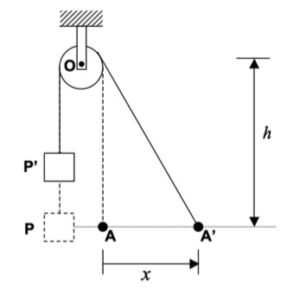# Homework H1.J - Sp23

 Problem statement Solution videoAsk your questions here. Or, if you can help someone by answering their questions, then do that here. You can learn either way.

DISCUSSION
This is a standard cable/pulley kinematics problem. You need to write the length, L, of the cable in terms of motion parameters for A and P. Let sP represent the distance that P is below the center of the pulley. From this, you can write: L = sP + sqrt(x^2 + h^2) + constant. Setting dL/dt = 0 gives you the relationship between sP_dot and x_dot.From the animation above, you can see that the speed of crate is never any greater than the speed of the worker, A. Can you see this result in your work?

## 19 thoughts on “Homework H1.J - Sp23”

1.Justin James Paluch says:

I got s_P dot to be a negative value which makes sense because the s_P is decreasing, but does the speed of the crate P have to be a positive value? If so, is that speed simply the derivative of h - s_P (the vertical position of P) with respect to t?

1.CMK says:

Justin: You are correct in getting s_P to be negative since the distance s_P is decreasing as A moves to the right. The way to phrase your answer is that "P is moving UPWARD with a speed of |s_P_dot|".

1.Justin James Paluch says:

Thank you!

2.Madeline B says:

I feel like I am missing something in my equation. I keep getting that s_P = - s_A but I feel like I should have the h and x affect this value, and I am not.

1.CMK says:

Madeline: The length of the cable section between O and A is given by sqrt(s_A^2 + h^2). I think that you must be saying that this length of simply s_A.

1.Madeline B says:

But would s_A equal 2 cause x=2? This is where I thought I would use the sqrt(x^2+h^2) but then I don't get an s_A or s_Adot variable.

1.CMK says:

x and s_A are interchangeable - they are different names for the same thing. If you take a time derivative of L = s_P + sqrt(x^2 + h^2) + constant, you will get the required relationship between s_Pdot and x_dot.

1.Nadra Elon Dunston says:

So, if we derived it correctly, there should be an x_dot (or s_A_dot) in the resulting equation?

3.Jenna says:

When taking the derivative of the length equation, you treat the height h as a constant right?

1.CMK says:

Yes, h is constant.

4.Colin Michael Porath says:

How is the length of rope wrapped around the pulley constant? Doesn't the angle of wrap change as the worker walks to the right?

1.Bradley Miles Kantor says:

I imagine the changing angle of wrap is negligible or we are at least ignoring it for this question.

2.Clairice Holmes says:

For these problems, we assume the pulley has a tiny negligible radius, so we can just ignore it’s effects.

5.Nadra Elon Dunston says:

When taking the derivative with respect to time of the L function, do I need to use the chain rule? None of the relevant variables are constant or in terms of other variables. So, I can derive directly, right?

1.Zachary William Delahunty says:

I did not use the chain rule for the L function. I don't believe it's necessary to solve the problem

2.Tate Peters says:

Actually, you do need the chain rule. This is a function of x and h f(x, h), so you do need to use the chain rule when solving for d/dt.

6.Anthony Michael Cialdella says:

My thought process was to solve the problem as if the new placement of the rope is the hypotenuse of a right triangle. Could you find the new length of the rope after 2 meters of lateral movement and also find the time it took to move that much? And then take the change of length of the rope divided by the time it took to get there which would equal the speed of the crate?

1.Myles Christian Kappes says:

I am not sure if this works, but I see the relationship you're talking about. The way I think about it is, that the bottom of the triangle is what is increasing with a certain velocity. This is different than if the hypotenuse is increasing with that velocity. If it were the hypotenuse increasing at the specified rate, then I believe it would be safe to assume that Vp and Va are the same. However, because it is the base of the triangle, they should not be the same, this can also be seen in the figure as delta_x is much larger than delta_P given the same time frame.

7.eaub says:

Is the expected answer a numerical value for |sp-dot|? If so, how can we determine the value of x-dot, is it simply just = Va?